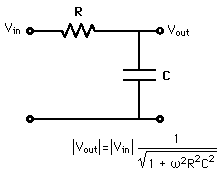# RC filtering circuits

Heya! I've got a lab practical tomorrow to do with RC circuits, what do the terminals at the top and bottom of a filter circuit diagram mean, I'm assuming Vin and Vout is the voltage in and then 0V/ground, but what do the bottom two terminals correspond to?Cheers

Heya! I've got a lab practical tomorrow to do with RC circuits, what do the terminals at the top and bottom of a filter circuit diagram mean, I'm assuming Vin and Vout is the voltage in and then 0V/ground, but what do the bottom two terminals correspond to?Cheers

The bottom left and right are the return only. $V_{in}\;$ is input voltage across the left two terminals from the top terminal to the bottom terminal on the left. $V_{out}\;$ is the output voltage across the right two terminals from the top terminal to the bottom terminal on the right.

Remember when you measure voltage, you need to measure between two terminals. You put the red probe on the top terminal and the black probe on the bottom terminal. Hope that help.